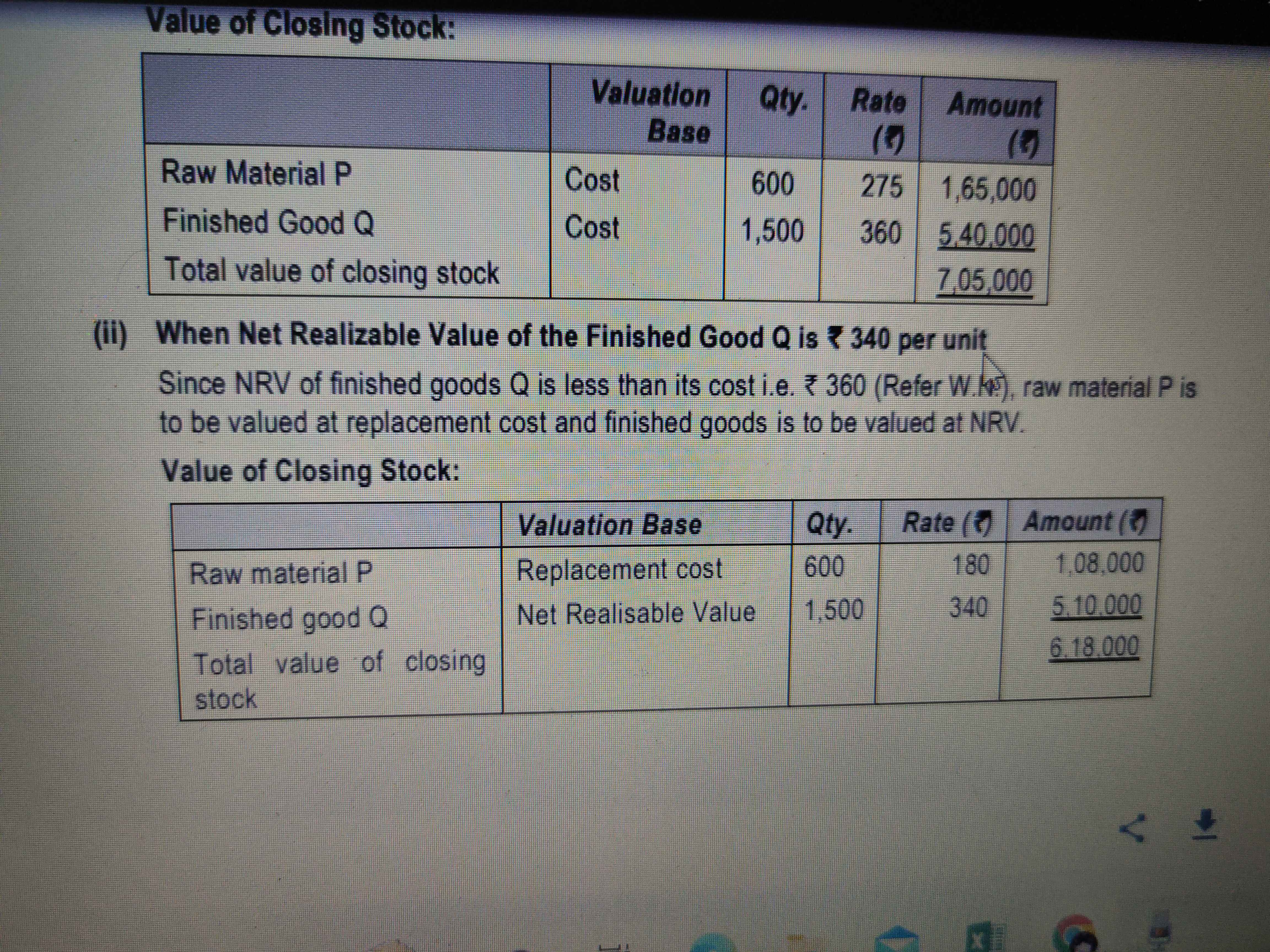Forums

# Raw material valuation

Accountancy

Suppose, FG NRV is 350, cost of FG is 360. Raw material cost is 140, while replacement cost is 190. What should be the value of raw material say I have 800 units. This ques is same as of Nov2019 PYP AS-2 question.Sugam SM

CA Inter

6K+

01-Dec-22

98

Value of raw material is Rs.140 being lower of cost or replacement costShankari C

CA Inter

12K+

01-Dec-22

So, 140*800 = 112000 is the valueShankari C

CA Inter

12K+

01-Dec-22

Shankari C

Value of raw material is Rs.140 being lower of cost or replacement cost

Where it is given in standard lower of cost or replacement costSugam SM

CA Inter

6K+

01-Dec-22

It is just said that if FG are sold below cost, then replacement cost to be taken for valuing raw materials.Sugam SM

CA Inter

6K+

01-Dec-22

Sugam SM

It is just said that if FG are sold below cost, then replacement cost to be taken for valuing raw materials.

You cannot value Raw Material above cost.Suraj Lakhotia

02-Dec-22

You cannot value Raw Material above cost.

So following prudence, it is not needed.Sugam SM

CA Inter

6K+

03-Dec-22

Then is it wrong?Sugam SM

CA Inter

6K+

03-Dec-22

Sugam SM

Then is it wrong?

Share the question. If replacement cost of raw material is less than cost, then repl.cost is taken. If greater, cost is taken.Shankari C

CA Inter

12K+

03-Dec-22

Sugam SM

Then is it wrong?

What is wrong?Suraj Lakhotia

03-Dec-22

Sugam SM

Then is it wrong?

It is right only. Replacement cost is less than cost.Shankari C

CA Inter

12K+

03-Dec-22

Sugam SM

Then is it wrong?

In this question FG cost is Rs.360 & its NRV value is Rs.340, i think u r confused regarding cost & NRV value!(Finished goods valued at NRV Being lower than the cost, then if Raw material replacement cost is also lower than cost, then RM valued at replacement cost)Yoganathan LAKSHMANAN

CA Inter

120

03-Dec-22

Sales